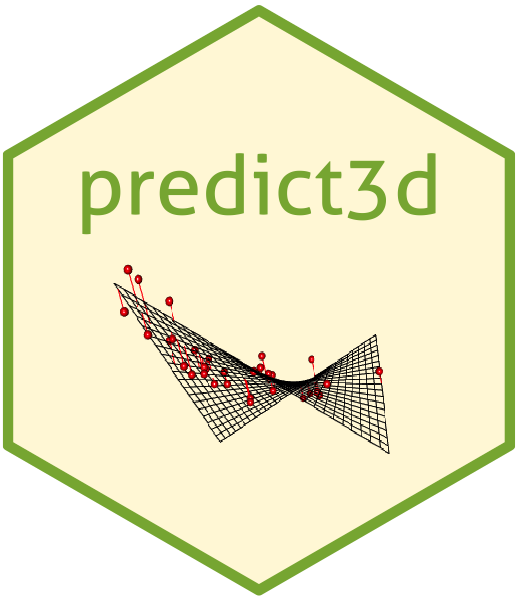# R package predict3dR package `predict3d` aims to draw predicts plot for various regression models. The main two functions are ggPredict() for 2-dimensional plot and predict3d() for 3-dimensional plot.

## Package Installation

You can install `predict3d` package from CRAN.

``install.packages("predict3d")``

You can install the developmental version of `predict3d` package from github.

``````if(!require(devtools)) install.packages("devtools")
devtools::install_github("cardiomoon/predict3d"")``````

## Linear Regression Models

You can draw linear regression models. First model has one categorical and one continuous explanatory variables.

``````require(predict3d)
require(rgl)
fit1=lm(Sepal.Length~Sepal.Width*Species,data=iris)
fit1

Call:
lm(formula = Sepal.Length ~ Sepal.Width * Species, data = iris)

Coefficients:
(Intercept)                    Sepal.Width
2.6390                         0.6905
Speciesversicolor               Speciesvirginica
0.9007                         1.2678
Sepal.Width:Speciesversicolor   Sepal.Width:Speciesvirginica
0.1746                         0.2110  ``````

You can draw plot for this model. ggPredict() function draws a scatterplot with regression line and shows regression equations parallel to the regression lines.

``ggPredict(fit1,digits=1)````````predict3d(fit1,radius=0.05)
rglwidget(elementId = "1st")``````You can see the live 3d plot at http://rpubs.com/cardiomoon/471347.

The second model has two continuous variables as explanatory variables. You can change the labels and the relative x position and the y position.

``````fit2=lm(mpg~wt*hp,data=mtcars)
ggPredict(fit2,labels=paste0("label",1:3),xpos=c(0.3,0.4,0.3))````````````predict3d(fit2)
rglwidget(elementId = "2nd")``````## Generalized Linear Models

You can draw generalized linear models.

``````require(TH.data)
fit3=glm(cens~pnodes*age*horTh,data=GBSG2,family=binomial)
ggPredict(fit3,se=TRUE,show.text = FALSE)````````````predict3d(fit3,radius=0.5)
rglwidget(elementId = "3rd")``````## Local Polynomial Regression Fitting

You can draw the loess model.

``````fit=loess(mpg~hp*wt,data=mtcars)
ggPredict(fit)````````````predict3d(fit,radius=2)
rglwidget(elementId = "4th")``````## Play with predict3d()

Once you have create a model with predict3d(), you can move your object with your mouse or R codes. For example, You can rotate you object with this R codes.

``````start <- proc.time()
while ((i <- 36*(proc.time() - start)) < 360) {
rgl.viewpoint(i, i/4);
}
play3d(spin3d(axis = c(1, 0, 0), rpm = 30), duration = 2)``````

You can save your 3d plot as a figure file or pdf file.

``````rgl.bringtotop()
rgl.snapshot("fig1.png")
rgl.postscript("fig2.pdf","pdf")``````

For more information about package `rgl`, please read the package vignette at: https://CRAN.R-project.org/package=rgl/vignettes/rgl.html

You can see the following vignettes published on rpubs.com

Drawing the multiple regression models(Part I)

Part II. Transforming variables